### Contravariance and Covariance - Part 2Josiah Willard Gibbs
Vector Calculus

Vector Concept 2 -- Vector Spaces
In the design of computer languages, the notion of contravariance and covariance have been borrowed from category theory to facilitate the discussion of type coercion and signature. Category theory, on the other hand, borrowed and abstracted the notion of contravariance and covariance from classical tensor analysis which in turn had its origins in physics.
In keeping with Feynman's observation that it is useful to have different ways thinking about known ideas, part 2 will be developing vectors from the point of view of linear algebra and vector spaces, which is a branch of algebra.
The axiomatic approach used in algebra requires the introduction of a number of definitions. However, only the definitions needed to illustrate contravariance and covariance in an abstract vector space will be given. So, unfortunately, for the sake of brevity, no detailed motivation of these mathematical concepts is going to be provided (*).
Needed Ideas From Linear Algebra
• Vector Space
• Linearly Independent
• Span
• Basis
• Coordinates
• Change of Basis
• Linear Functional
• Dual Space
The definition of a vector space also uses the algebraic concept of a field. (Not to be confused with a physical field, like, for example, the gravitational field...same words, different concepts.) For those who have not studied fields and do not wish to, good examples are the real numbers R, or the complex numbers C, which can be substituted in the definition below to allow you to follow along. Boldface is used to indicate elements of the vector space, normal font is used to indicate scalars
We now introduce some notation, and a convention, that will be useful. The Kronecker delta is defined by: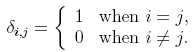Also, the Einstein summation convention will be used. That is, whenever repeated indices appear in an equation, it is understood that a summation is taken over these repeated indices.
##### Vector Space
A vector space V consists of a set of objects, called vectors, and a field F, called scalars , and a binary operation +  on vectors, that satisfies the following axioms:

i) if a and b are vectors, then so is a +b for all vectors a and b
ii) a+ b =b + a
iii) a + (b + c) = (a + b) + c
iv) There is a zero vector, 0, such that 0 + a = a + 0 = a for any vector a
v) There are additive inverses. For each vector a, there exists a vector -a such that a + (-a) = -a + a = 0.
vi) Scalar multiplication. For any scalar k and any vector a, k a is a vector.
vii) k(a + b) = ka + kb
viii) (k + l)a = ka + la for any scalars k and l
ix) k(l(a)) = kl(a) for any scalars k and l
x) 1a = a1 = a where 1 is the scalar identity

##### Linear Independence
A set of vectors {v1,...,vk} is linearly independent iff
a1v1 + ... + akvk = 0 implies a1=...=ak= 0

##### Span
The span of a set of vectors {v1,...,vk} is the intersection of all subspaces that contain {v1,...,vk}

A straightforward result is that the span of a set of vectors {v1,...,vk} is given by all possible linear combinations of the {v1,...,vk}. This result is often more useful to work with than the actual definition.
##### Basis
A basis for a vector space is any set of linearly independent vectors that span V.

Given a basis for V, the number of vectors in the basis is called the dimension of V. A finite dimensional vector space is a vector space that is spanned by a finite number of vectors. The discussion will be limited to finite dimensional vector spaces.
##### Coordinates
Let v be a vector in V, and let β = {v1,...,vn} be an ordered basis for V. Then if v = a1v1 + ... + anvn , the scalars a1,...,an are called the coordinates of v with respect to the basis β, and is written as a nx1 column matrix: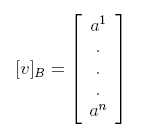##### Change of Basis
We do not wish to be constrained to one basis. If we formulate an equation that uses coordinates in its formulation, we would like to know that it is true independent of the basis chosen. Therefore, we need to know how to express our equation in a new basis. Also, it is often the case that we are able to transform to a new basis where our equations take on a simpler form. For these reasons, it important to study the change of basis problem.
Let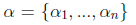be a basis for V and v be an arbitrary vector with coordinates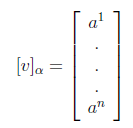If we now instead wish to use a different basis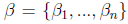how do we calculate the new coordinates?To answer  this question, expand v in the beta basis, using the cordinates just given:Then, writing this in coordinate form in the alpha basis gives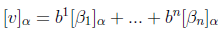This can be re-written as a nxn matrix times a nx1 matrix as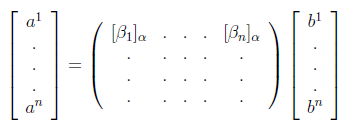where the nxn matrix's columns are the coordinates of the beta basis vectors with respect to the alpha basis. Denoting the nxn matrix, by P, this can be written as
vα = P vβ
and left multipling by P-1 gives the require expression for the coordinates of v in the new basis:
vβ = P-1 vα
The matrix P is called the transistion matrix from the α basis to the β basis.
( A word on convention: if instead of expanding v in the β basis above, I had expanded v in the α basis, and taken coordinates in the β basis, I would have finally arrived at a slightly different form:
vβ = Q vα
where Q is the matrix formed by the coordinate vectors of the α basis vectors with respect to the β basis. Occasionally, people call Q the transition matrix. If you are reading a book on linear algebra you need to check the convention being used.)
Having provided most of the needed definitions, we are very close to having all language needed to state the main idea. Recall in the "directed arrow" definition of a vector,  the contravariant components of a vector were its coordinates with respect to the reciprocal lattice, and the reciprocal lattice was defined by the requirement ei.ek = 0. In the abstract vector space formulation, this notion is generalized by the concept of the dual space and dual basis, which we now introduce.
##### Linear Functional
Definition: A linear functional on a vector space V is a linear map from V into the scalars K.

##### Dual Space and Dual Vectors
The set of all linear functionals on a vector space V is called the dual space of V, denoted by V*. Elements of the dual space are called dual vectors .

If V is a vector space of dimension n, then V* is a vector space of dimension n. Given a basis α for V, we construct a basis for V* as follows:
##### Dual Basis
For a given basis α,the set of linear functionals,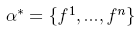defined byis a basis for V*, called the dual basis of α .

We now know how to get a basis for V* given a basis for V . This allows us to write the components for an aritrary dual vector. We now want to enquire how the coordinates of our arbitary dual vector change when a change of basis is performed is performed in V. The change of basis induces a change of basis on the dual space.
 Old Bases New Bases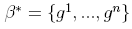The key to discovering how dual vectors transform is to require that the new dual basis vectors, gi, satisfy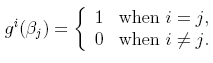Let the transformation matrix to the new dual basis be hik . Then gi=hik fk, where the Einstein summation convention is used to indicate a summation is taken over the indices k. If βj = Qjlαl then we have
hik fkQjlα l   = δij
Using the summation convention and simplifying this reduces to
hik Qjl   δkl = δij
and simplifying one more time gives
hil Qjl= δij
or in other words
hil QTlj= δij
where QT is the transpose of Q.
Thus we have the important result that
h = (QT)-1
So, the coordinates in the new dual basis are computed from the change of basis matrix by taking the transpose inverse of the transformation matrix and using that as the transformation matrix for the dual vectors. The dual vectors are also called contravariant vectors, and the vectors in the original space are called covariant vectors. The contravariant vectors are said to transform contragrediently and the covariant vectors transform cogrediently.
Next week -- Tensor analysis and vector transformation laws.

(*) Starting with part 2, the discussion becomes more abstract. I cover quickly what is usually covered at a more leisurely pace in advanced linear algebra coures. I recommended "Linear Algebra" by Kunze and Hoffman as a reference, and also Schaum's Linear Algebra for practice problems.
There are other possible reference books (for example, Strang's unfortunate text "Linear Algebra and Its Applications") on linear algebra, but they devote a lot of time to other topics before even getting to linear transformations, which is the heart of the linear algebra. Kunze and Hoffman is the standard reference. The problem with Strang is a student can take a linear algebra course using his text, and not understand the centrality of linear transformations because Strang spends so much time on other, and easier, topics. Solving equations using matrices with either matrix multiplication , or Gaussian elimination techniqes, which Strang devotes much time to is rather trivial. In fact, these topics I have successfully taught to a 9 year old. Vector spaces, basis, and linear transformations is more demanding.